Transcripted Summary

We already have quite a few tests.

Now, in some situations, we might want a test method not to execute based on some conditions that can be evaluated into a boolean.

So based on the condition, which may result in true, we don't want the test to be run, but we don't want it to fail either.

So we want to kind of skip the test based on that condition.

Here, we can use JUnit 5's assumption features, which will allow us to skip either an entire test or parts of the test based on the conditions that we will provide to the so-called Assumptions.

Let's take a look.

I will start looking at assumptions by creating a new test class.

I will just call it `AssumptionsTest`.

I will go to my `ParameterizedTests` class, and I will just copy-paste one of the methods we created here - namely the first one, the `intValues` one.

I will use this one as the first example, and I will copy and paste it to the `AssumptionsTest` class.

So here, as you can see, we have a `@ParameterizedTest`, which takes three values: 1, 5, 6. These are int values.

Let's run the test and see that the test actually runs.

So yes, we do have three test runs here.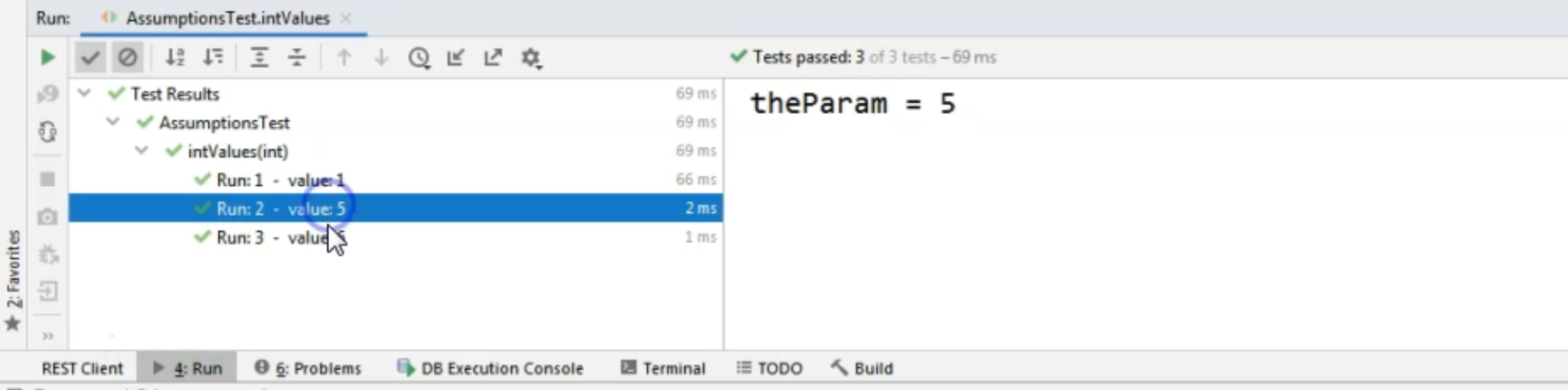### # Assumptions, what are they?

I mentioned in the intro that based on a condition which evaluates to boolean, we can skip the run of certain tests.

I will give a quick example in this method.

We have several assumptions available.

They can be found in the Assumptions class in `org.junit.jupiter.api`, and they are: `assumeFalse`, `assumeTrue`, and `assumingThat`.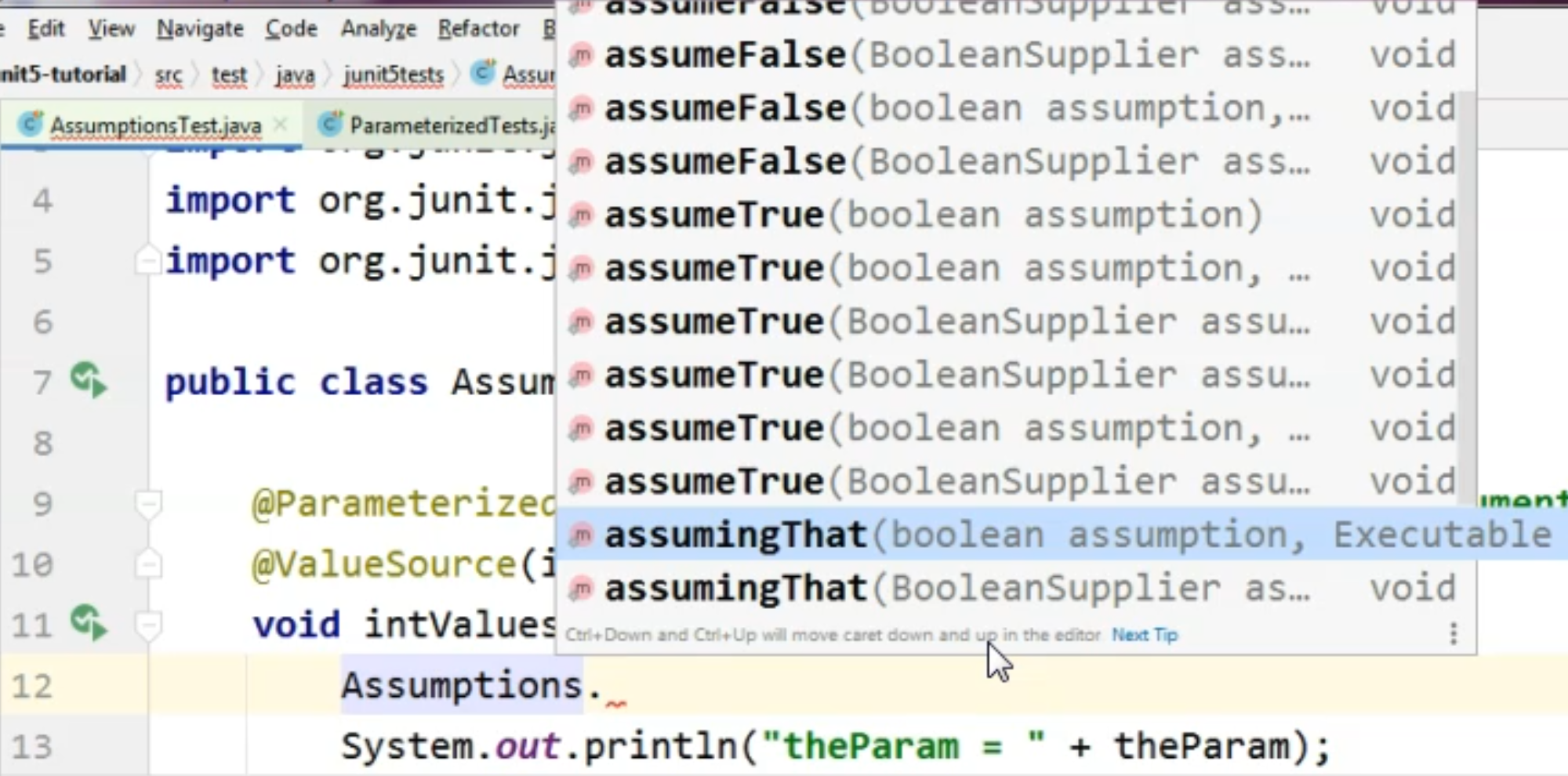They come in different flavors, so they have different signatures.

### # Assumptions.assumeTrue

The most basic one takes a condition which evaluates to boolean, and then we also have the option to provide a message that will be shown at the console when the test will be skipped.

But let's try with a very simple example.

Let's just say `Assumptions.assumeTrue`.

In order to see how the test will run when we are using Assumptions, I will just pass as the condition to this assumption that `theParam > 4`.

I will also do a static import here.

So now our test starts with `assumeTrue` that the param is greater than four.

`assumeTrue` means that if the condition provided to the assumption evaluates to true, only then will the test run. If the condition does not evaluate to true, the test will be skipped.

As we can see, our parameters that are passed through the test method are 1, 5, 6, and ` is the only parameter that doesn't satisfy the condition from the assumption.

Therefore, we expect the test method not to run with the value 1, but instead to skip that test run.

``````package junit5tests;

import org.junit.jupiter.api.Assumptions;
import org.junit.jupiter.params.ParameterizedTest;
import org.junit.jupiter.params.provider.CsvSource;
import org.junit.jupiter.params.provider.ValueSource;

import static org.junit.jupiter.api.Assumptions.*;

public class AssumptionsTest {

@ParameterizedTest(name = "Run: {index}  -  value: {arguments}")
@ValueSource(ints = {1, 5, 6})
void intValues(int theParam) {
assumeTrue(theParam > 4);
System.out.println("theParam = " + theParam);
}
}
``````

Let's see how this happens.

Let's rerun the test method and see the new result in the console.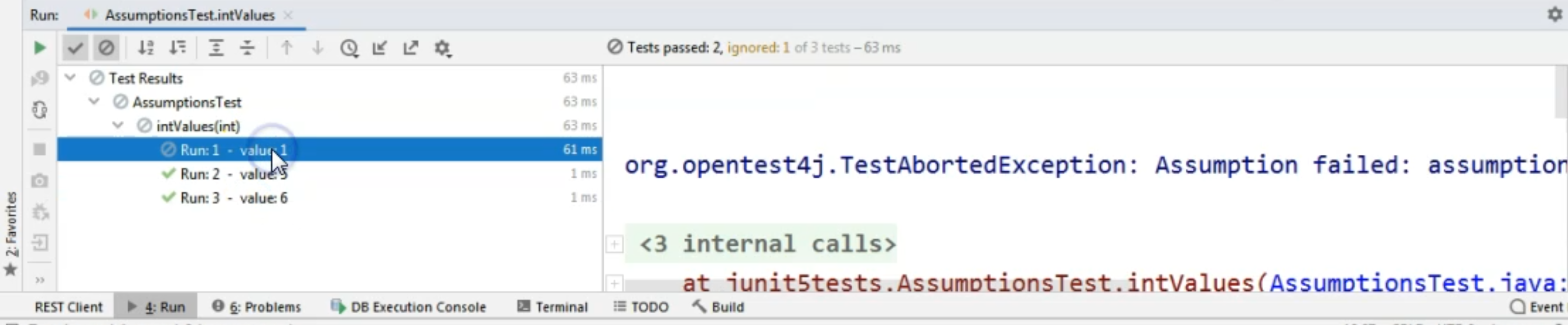As you can see, the second and third methods ran properly, but the first one was skipped.

It was skipped with a so-called exception, but it did not fail.

So there's a special type of error here, and it just says that the Assumption failed - the assumption was not true.

As you can see, this message is not necessarily the best one, so it doesn't necessarily give us the best information.

So we could create another assumption where we can provide a message to be displayed to the console when the test is run.

### # Assumptions.assumeFalse

Let's go back and copy a different method from all of these that we have available here.

So let's take, for example, this method where we have passed the names of our favorite superheroes, and let's paste this one into our `AssumptionsTest` class.

Here I will do an assumption, and I will say `assumeFalse` - so let's try a different type of assumption her - and I will say `assumeFalse(param1.equals("steve"))`, and if this assumption will fail, we want the following message to be printed to the console - namely, "The assumption failed for the following param2."

We want to know which was the value for `param2` when this test was skipped.

``````    @ParameterizedTest
@CsvSource(value = {"steve,rogers", "captain,marvel", "bucky,barnes"})
void csvSource_StringString(String param1, String param2) {
assumeFalse(param1.equals("steve"), "The assumption failed for the " +
"following param2: " + param2);
System.out.println("param1 = " + param1 + ", param2 = " + param2);
}
``````

As you can see, we have `assumeFalse` that `param1` equals "steve".

Because we don't want `param1` to be "steve", the tests that we'll run will use the second and the third set of parameters.

We can demonstrate that by running the test for the first time and seeing that the first test run was aborted.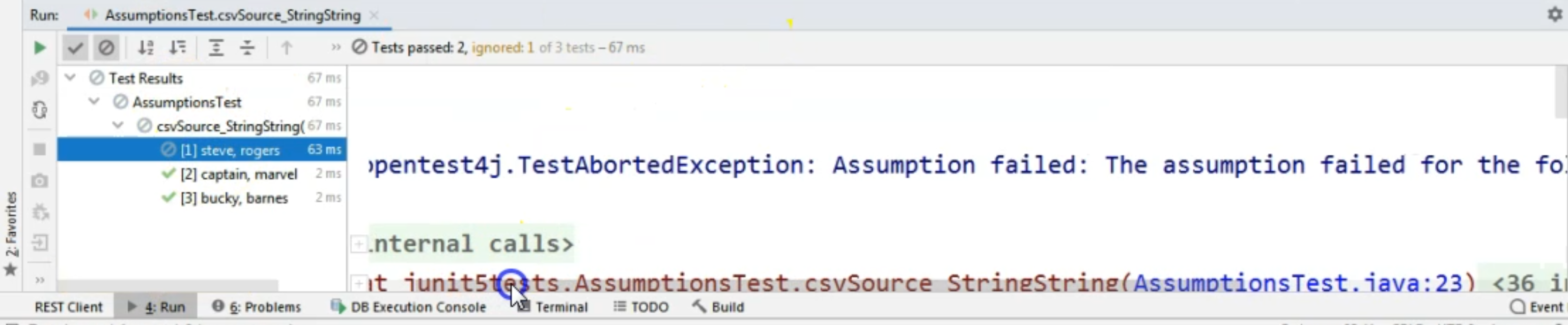The message that we got in the console is that the "assumption failed for the following param2: rogers".

The total number of tests run in this case is three - two were passed, as you can also see in the left-hand side, and one was ignored.

### # Assumptions.assumingThat

Let's take a look as a third and final example for this chapter, and let's go back to the `ParameterizedTests` and copy the method where we have three parameters - a string, an int, and a boolean.

I will copy this one and I will paste it back in our `AssumptionsTest` class.

Here let's try out a different assumption - namely, the `assumingThat`.

Here what I want to do is, based on the condition, only skip a part of the test.

So I just want to create some code to be executed within this assumption, and it should be executed only when the condition that I will supply to the assumption is true.

Taking a look at the parameters that I have available, I want to create an assumption where I will take a look at the value for `param2`.

I will say that only when `param2 > 20`, I want to do a `System.out` to the console to say that "This code ran."

So I want to signal that the code inside the assumption ran.

In this case, when we're using `assumingThat`, and we are specifying the code to be executed inside the assumption, the rest of the test will run no matter what the result of evaluating this condition from within the assumption will be.

The assumption, in this case, is independent of the rest of the code in the test.

This is different from what we had in `assumeFalse`, for example.

Here, we will not skip the entire test if `param2` is lower than `20`, but instead we will skip doing this `System.out` if `param2` is lower than `20`.

``````    @ParameterizedTest
@CsvSource(value = {"steve,32,true", "captain,21,false", "bucky,5,true"})
void csvSource_StringIntBoolean(String param1, int param2, boolean param3) {
assumingThat(param2 > 20, () -> System.out.println("This code ran"));
System.out.println("param1 = " + param1 + ", param2 = " + param2 + ", param3 = " + param3);
}
``````

Let's run the test method and see the result.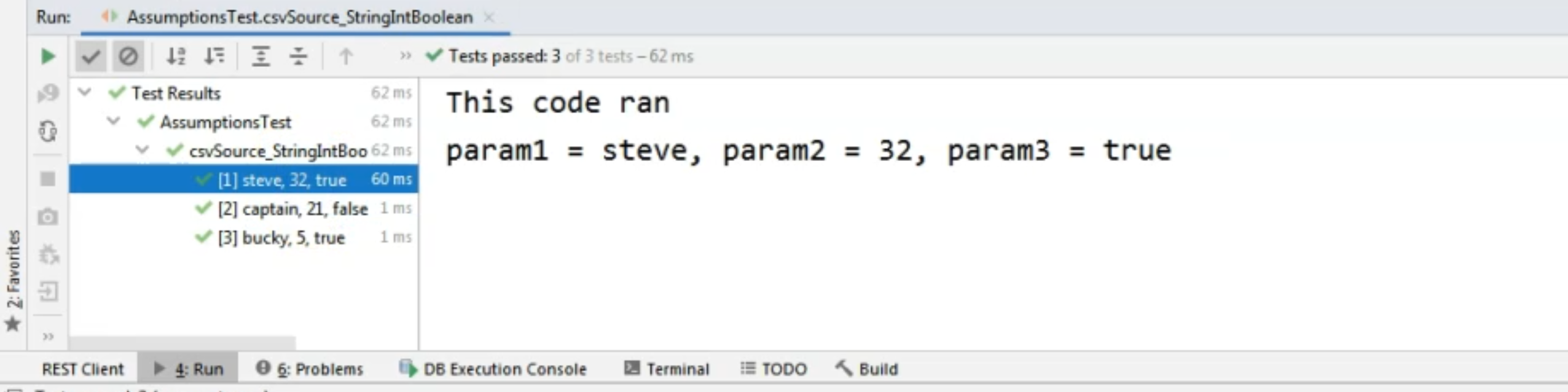This time we will have three method runs as you have seen here, even though for "bucky" we have the value "5" for "param2".

Now for the third run, we don't have the "This code ran" system output.

However, if we're looking at the others, we will see that the code from within the assumption did run successfully because the condition was met for "param2" in both these cases.

So this is how we can use the assumption to skip a part of the test, and in the previous two methods, we have seen how to skip a test entirely if that is what we need.

Assumptions Require A Condition

The important thing to remember here is that within the assumption, we need to provide a condition to be evaluated, no matter what that condition is, and that it needs to evaluate to a boolean value.

For example, we might look for some system properties, we might need to check some sort of data.

No matter what checks we are doing, let's return a boolean, and then based on the boolean, we can skip or not skip the test method run.